### Epsilon#### THE EPSILON LEVEL FOCUS: Fractions, Factors, Mixed Numbers and more

##### Major Concepts and Skills:
• Recognizing and generating equivalent fractions
• Understanding addition, subtraction, multiplication, and division of fractions and mixed numbers
• Fluently adding, subtracting, multiplying, and dividing fractions and mixed numbers
• Using multiple strategies to recognize common factors
• Understanding grouping symbols and their effect on order of operations
• Interpreting and solving word problems
• Comparing, and converting decimal fractions
• Finding the area and circumference of circles
• Representing fractions and fractional measurements on line plots and number lines
• Using coordinates to represent ordered relationships
& more …

##### Purchase Epsilon Complete Set
• 1 x Fraction Overlays Kit
• Epsilon Student Kit
• Epsilon Instruction Manual### \$399.00

+ GST

\$399.00 ex. GST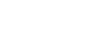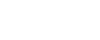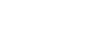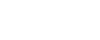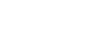#### Express shipped to youFraction Overlays Kit
1 x Fraction Overlays Kit

The Fraction Overlays show fractions of any number in in a hands-on, multisensory way. This is teachers' favourite tool - making it easy to understand for any student.Epsilon Instruction Manual:
1 x Epsilon Instruction Manual (with Solutions)

This manual will provide you with step-by-step instructions on how to teach each lesson to your student. This manual should be used in collaboration with the video lessons.Epsilon Student Kit:
1 x Epsilon Student Text
This workbook gives your student extra practice on what they've been taught from the Instruction manual and video lessons - and should be used in conjunction with the Fraction Overlays Kit.

#### Instant Online Access12 Months Epsilon Member Access

Bonus Resources:
Scope and Sequence of each lesson
Lesson Objectives  Sheets
Online Completion Certificates
+ more

Ongoing Support:
Basic Training & Initial Setup
Phone & Online Support##### The Epsilon Complete Set includes:
• 1 x Fraction Overlays Kit
• Epsilon Student Kit
• Epsilon Instruction Manual

### \$399.00

+ GST

\$399.00 ex. GST##### Want to purchase your level materials separately?Fraction Overlays Kit

### \$99.00

+ GST

\$99.00 ex. GSTEpsilon Instruction Manual
+ 12 Months video access

### \$299.00

+ GST

\$299.00 ex. GSTEpsilon Student Kit

+ GST

\$79.00 ex. GST